Open in App
Not now

# Image Formation by Lenses

• Last Updated : 16 Nov, 2022

In optics, a ray is a geometrical representation of the light that is idealized by choosing a curve that is perpendicular to the wavefronts of actual light and points in the energy flow direction. Rays are used to represent the propagation of light through an optical system by separating the real light field into discrete rays that can be computationally carried through the system using ray-tracing techniques. This makes it possible to investigate or simulate even the most complex optical systems mathematically. Ray tracing is based on approximate solutions to Maxwell’s equations that hold true as long as light waves flow through and around objects with dimensions significantly greater than the wavelength of the light. Diffraction, for example, necessitates the study of wave optics, which is not addressed by ray or geometrical optics. Adding phase to the ray model can be used to describe wave phenomena such as interference in some instances.

## What are Lenses?

Light beams flow through lenses, which are optical devices that allow light to pass through them. A lens is a transparent substance constrained by two curved surfaces that can refract light rays. A lens can have one surface plane and another spherical surface, indicating that it has at least one.

Lenses are often made of a thin piece of glass or plastic. Binoculars, telescopes, vision-correcting spectacles, torches, and microscopes are just a few examples.

## Types of Lenses

Depending on whether light rays are converging or diverging, there are two types of lenses. There are two types of lenses:

1. The convex lens (converging lens)
2. The concave lens (diverging lens)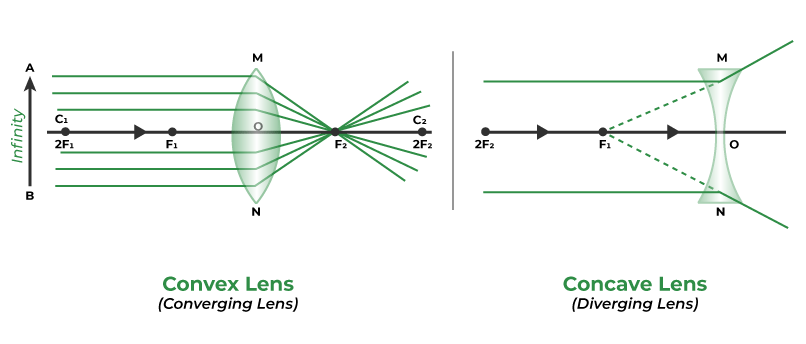### Concave lens

The lens which is thicker at the end than the middle is called the concave lens. It is also called diverging lens as it spreads out the light rays that have been refracted through it. It has the ability to diverge the parallel beam of light.

A common application of concave lenses is that they are used in optical devices such as binoculars, telescopes, eyeglasses, spy holes in doors, etc.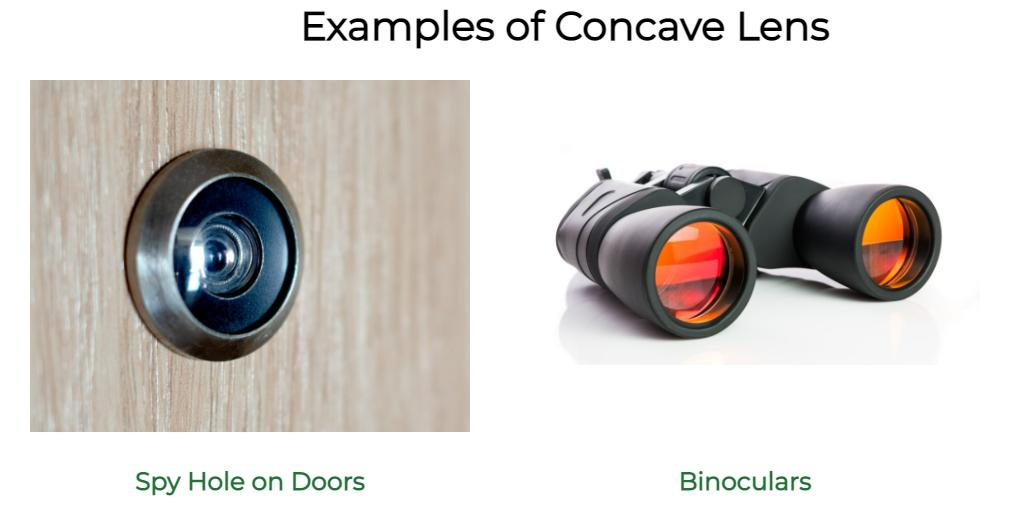### Convex lens

The lens which is thicker at the middle than the end is called a convex lens. It is also called a converging lens as it converges the parallel beam of light into a point.

A common application of convex lenses is that they are used in optical devices such as microscopes, telescopes, eyeglasses, magnifying glasses, etc.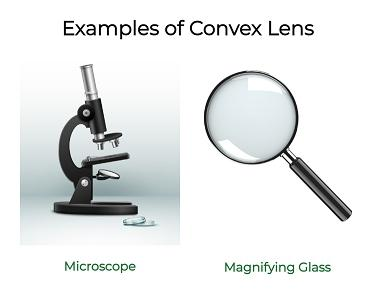## Terminologies Related to Spherical Lens

• Pole (p): It is the middle point of the spherical lens or mirror.
• Centre of curvature (C): It is the center of the sphere from which the mirror is formed.
• Principal axis: It is the lines passing through the pole and the center of curvature of the lens.
• Principal focus (F): It is the point at which a narrow beam of light converges or diverges.
• Focal length (f): It is the distance between the focus and the poles of the mirror.

## Image formed by the Convex Lens

There are six different cases for image formation by a convex lens, which are discussed as:

### When an Object is at Infinity

When object AB (shown in the figure below) is placed at infinity that is behind the 2F1 of the convex mirror, the image formed after the refraction will on focus F2 which is on the opposite side of the convex lens. The size of the image is smaller than the object and the image will be real and inverted(i.e upside down and downside up).

• The image formed at – Focus (F2)
• The nature of the image formed – Real and inverted
• The size of the image formed – Diminished (smaller)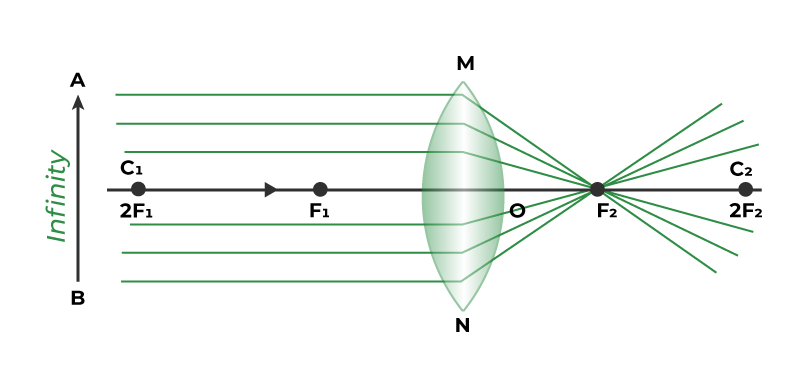### When an Object is placed Behind the Centre of Curvature (C1)

When the object is placed behind the center of curvature (C1) or behind the Focus (2F1) of the convex lens, the image formed after the refraction will be between the foci of another side of the lens (i.e. F2 and 2F2). The size of the image is smaller than the object. The nature of the image will be real (can be seen on the screen) and inverted( upside down).

• The image formed at – Between 2F2 and F2.
• The nature of the image formed – Real and inverted
• The size of the image formed – Diminished (smaller)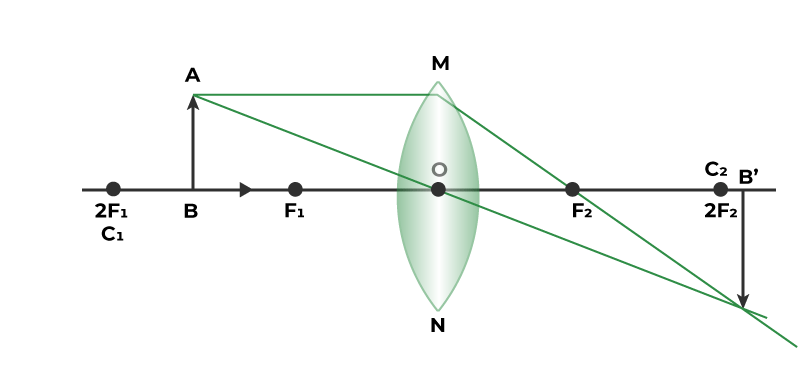### When the Object is placed at the Center of Curvature (C1 or 2F1)

When an object is placed at the center of curvature (C1) or focus (2F1) of the convex lens, the image formed after the refraction will be on the center of curvature (C2) or focus (2F2) on the other side of the lens. The size of the image is the same as the size of the object. The nature of the image is real and inverted.

• The image formed at – C2 or 2F2.
• The nature of the image formed – Real and inverted
• The size of the image formed – Equal to the object size.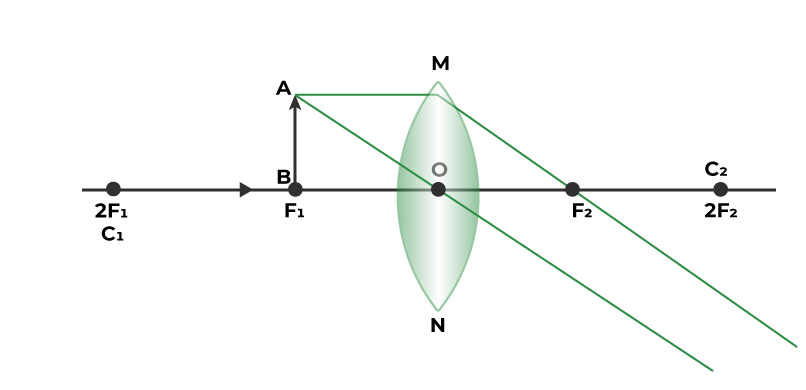### When the Object is placed between 2F1 and F1

When an object is placed between the center of curvature and the focus (F1) of the convex lens, the image formed after reflection will be behind the center of curvature (C2). The size of the image will be greater than the object. The nature of the image will be real and inverted.

• The image formed – Behind the center of curvature (C2)
• The nature of the image formed – Real and inverted
• The size of the image formed – Enlarged### When the Object is placed at Focus (F1)

When an object is placed at focus (F1) of a convex lens. The image formed after reflection will be at infinity (opposite side of the lens). The size of the object will be much larger than the object. The nature of the image will be real and inverted.

• The image formed at – Infinity (opposite side of the object)
• The nature of the image formed – Real and inverted
• The size of the image formed – Enlarged### When the Object is placed between Pole and Focus (O and F1)

When the object is placed between the focus (F1) and the optic center (O) of the convex lens. The image is formed at the same side of the object behind the center of curvature (C) or focus (F1) of the lens. The size of the image will be larger than the object. The nature of the image will be Virtual Erect.

• The image formed – At the same side of the object behind 2F2.
• The nature of the image formed – Virtual and Erect.
• The size of the image formed – Enlarged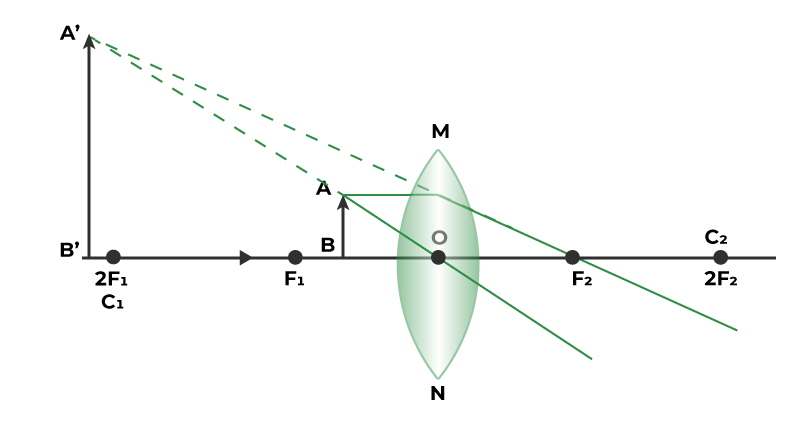## Image formed by Concave lens

There are only two different cases for image formation by a concave lens, which are discussed as:

### When the Object is placed at Infinity

When an object is placed at infinity of the concave lens (shown below). The image formed after refraction will be at the focus (F1) on the same side of the object. The size of the image will be much smaller than the object. The nature of the image will be virtual and erect.

• The image formed at – Focus (F1)
• The nature of the image formed – Virtual and Erect
• The size of the image formed – Highly diminished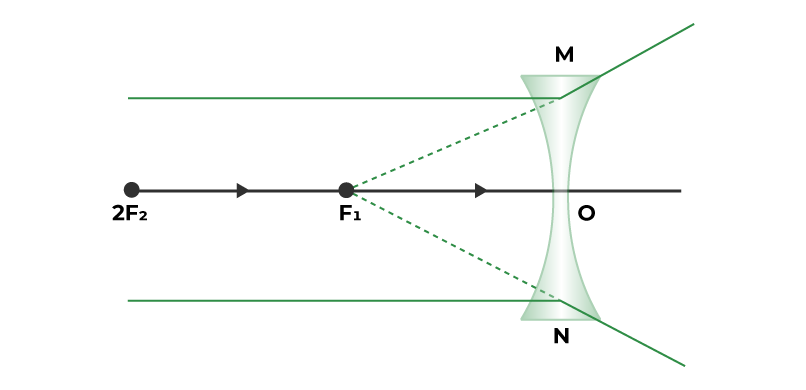### When the Object is placed at a Finite Distance from the Lens

When the object is placed at any finite distance in front of the concave lens. The image formed after refraction will be between the optic center (O) and the focus (F) of the concave lens. The size of the image will be smaller than the object.

• The image formed at – Between F1 and the optical center
• The nature of the image formed – Virtual and Erect
• The size of the image formed – Diminished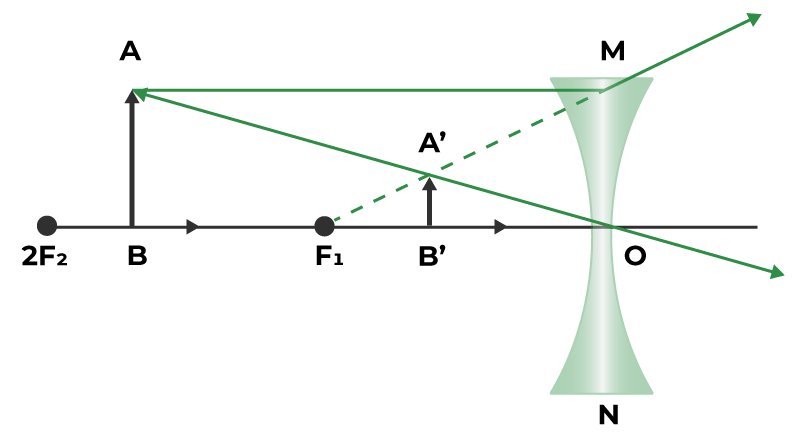## FAQs on Image Formation by Lenses

Question 1: What is the real image?

The image formed when rays of light meet at a certain point after reflection/refraction is real image. Real images can be displayed on screen.

Question 2: What is a virtual image?

The image formed when rays of light appear to meet at particular point is called virtual image.

Question 3: What is a ray diagram?

The type of diagram which helps to trace the path that light takes in order for a person to view a point on the image of an object is called a ray diagram.

Question 4: Which lens would you use as a magnifying glass?

Convex Lens is the lens which is used in the magnifying glass.

Question 5: What will be the focal length of a lens when it is cut along the principal axis?